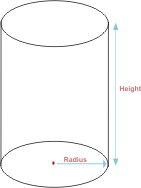## 圆柱体积计算器

 输入数值： 单位： Inches Metres Centimetres Millimetres Yards Feet 半径： 高： 结果： 圆柱体积：1、柱体（包刮棱柱、圆柱）：V=S底*h S表=2S底+Lh

2、椎体（圆锥）：V=(1/3)S底*h S表=底面圆半径*母线长*π （棱锥）：V=(1/3)S底*h S表=S侧+S底

3、圆台：V=(1/3)(S1+√(S1S2)+S2)*h （注：S1=上底面积 S2=下底面积 h=圆台高）

4、球体：V=(4/3)πr^3 S表=4πr²